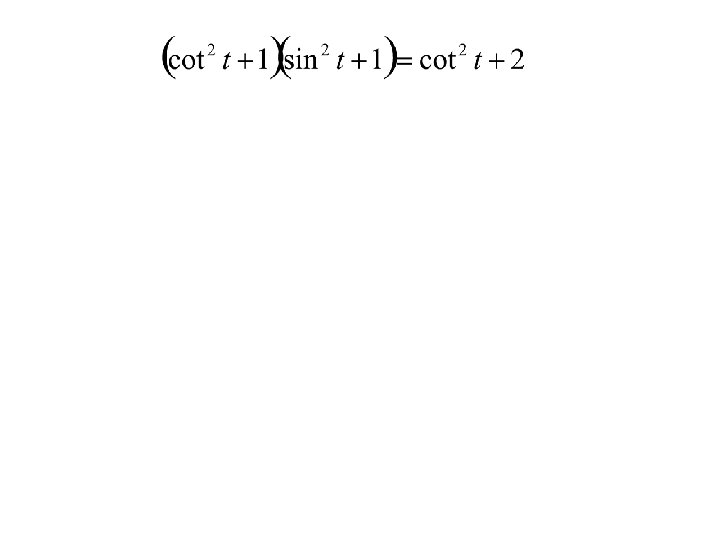# Verifying Trigonometric Identities Basic Trigonometric Identities Text Example

• Slides: 11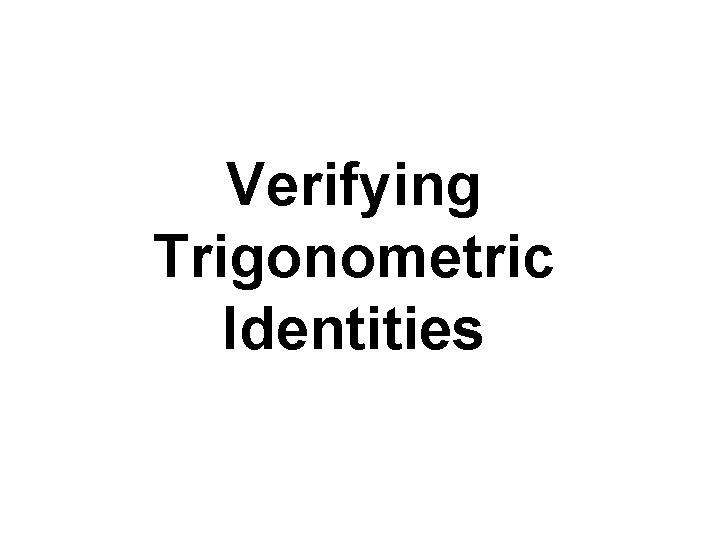Verifying Trigonometric IdentitiesBasic Trigonometric IdentitiesText Example Verify the identity: sec x cot x = csc x. Solution The left side of the equation contains the more complicated expression. Thus, we work with the left side. Let us express this side of the identity in terms of sines and cosines. Perhaps this strategy will enable us to transform the left side into csc x, the expression on the right. Apply a reciprocal identity: sec x = 1/cos x and a quotient identity: cot x = cos x/sin x. Divide both the numerator and the denominator by cos x, the common factor.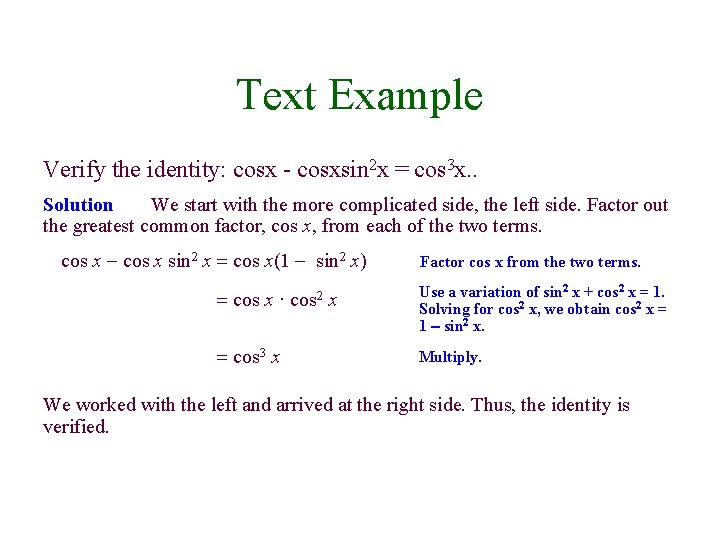Text Example Verify the identity: cosx - cosxsin 2 x = cos 3 x. . Solution We start with the more complicated side, the left side. Factor out the greatest common factor, cos x, from each of the two terms. cos x - cos x sin 2 x = cos x(1 - sin 2 x) = cos x · = cos 3 x cos 2 x Factor cos x from the two terms. Use a variation of sin 2 x + cos 2 x = 1. Solving for cos 2 x, we obtain cos 2 x = 1 – sin 2 x. Multiply. We worked with the left and arrived at the right side. Thus, the identity is verified.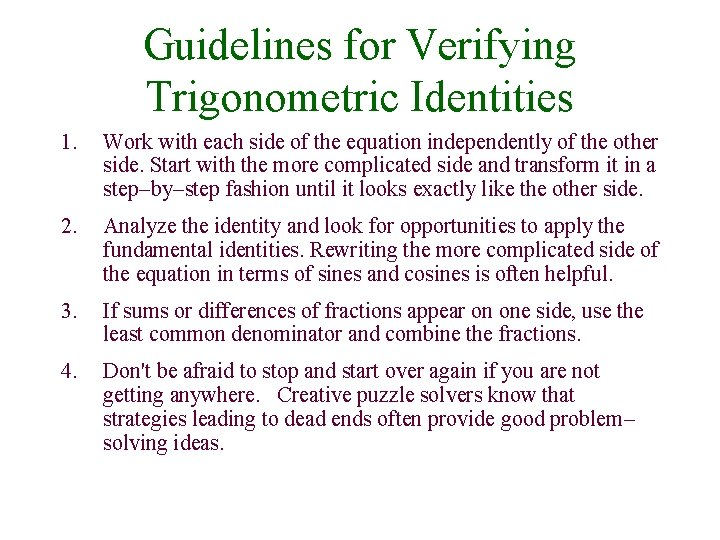Guidelines for Verifying Trigonometric Identities 1. Work with each side of the equation independently of the other side. Start with the more complicated side and transform it in a step-by-step fashion until it looks exactly like the other side. 2. Analyze the identity and look for opportunities to apply the fundamental identities. Rewriting the more complicated side of the equation in terms of sines and cosines is often helpful. 3. If sums or differences of fractions appear on one side, use the least common denominator and combine the fractions. 4. Don't be afraid to stop and start over again if you are not getting anywhere. Creative puzzle solvers know that strategies leading to dead ends often provide good problemsolving ideas.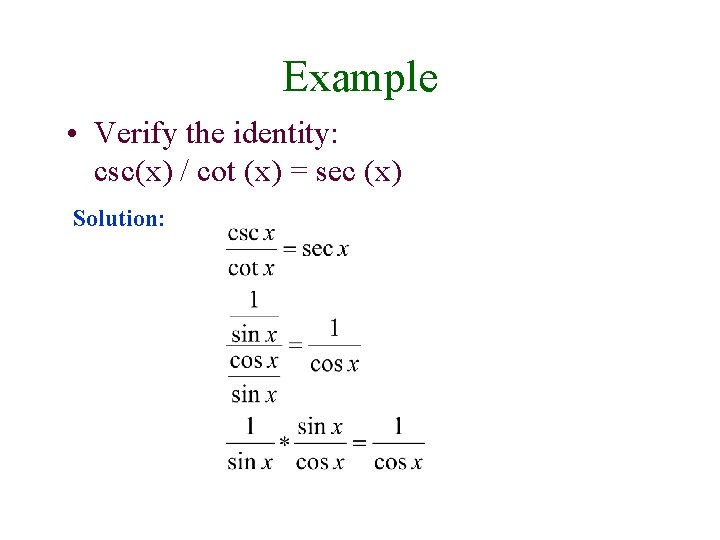Example • Verify the identity: csc(x) / cot (x) = sec (x) Solution: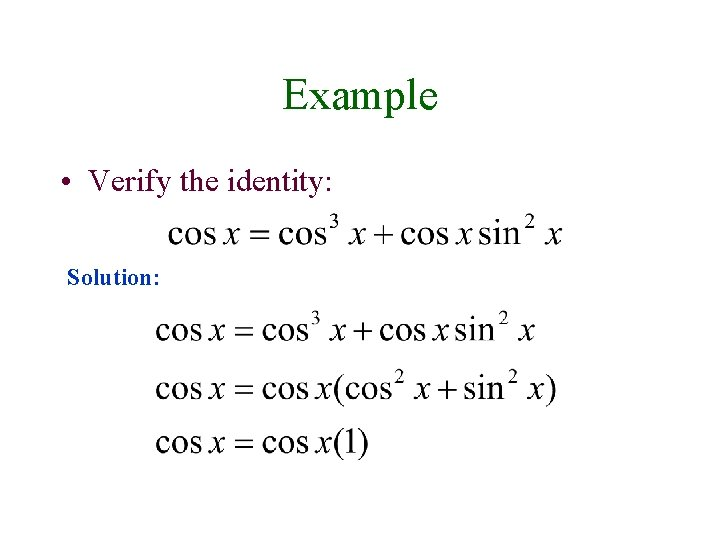Example • Verify the identity: Solution: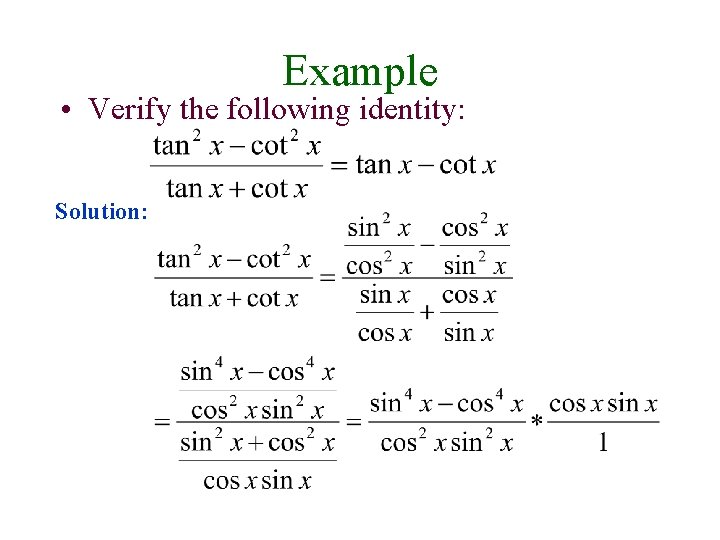Example • Verify the following identity: Solution: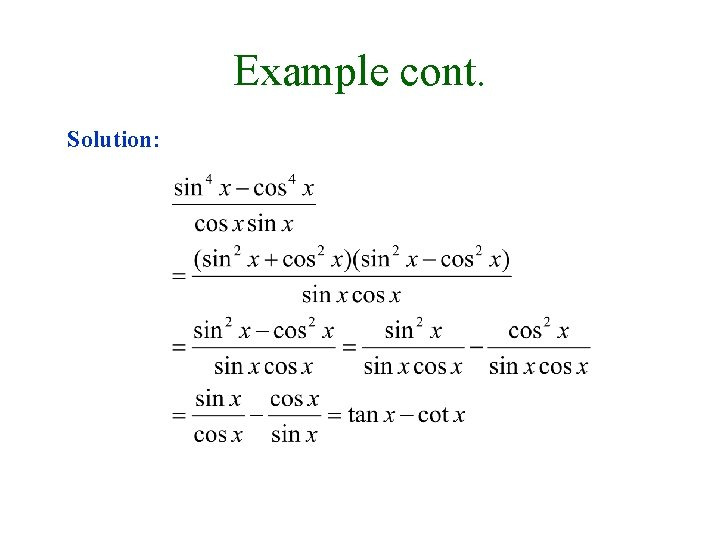Example cont. Solution: